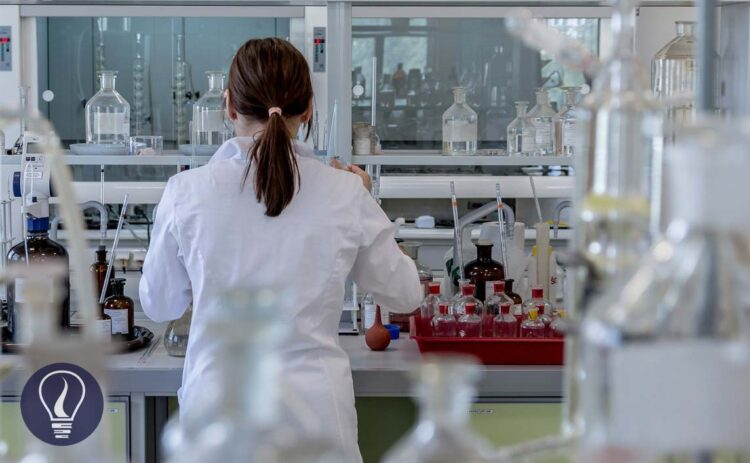More results...

Generic selectors
Exact matches only
Search in title
Search in content
Post Type Selectors

# How to Calculate the Hardness of Water by EDTA TitrationB.Sc (Hons, USJ) (Polymer Science and Technology, Chemistry, Physics)
Categories
Last Updated On : August 6, 2023
Published Date : February 24, 2023

## The Hardness of Water

In general definition, the hardness of water is described as a measurement of the capacity of water to precipitate soap. Water hardness level is a parameter that is very important in water quality analysis.

Soap is mainly precipitated by Calcium ions and Magnesium ions. Also, some other polyvalent cations also have the ability to precipitate soap. But those cations are normally in a complex form with organic compounds. Therefore, their role in the hardness of water is negligible and very difficult to measure.

So, the total hardness of water is defined as the total amount of calcium and magnesium ion concentrations. Both are stated as calcium carbonate, in milligrams per liter unit. Hardness water samples are determined by EDTA Titrimetric Method.

Other than the total hardness of water there is “Carbonate hardness”. Carbonate hardness is also known as carbonate and bicarbonate alkalinity. In carbonate hardness, we measure the milligrams of carbonate and bicarbonate ions dissolved in one liter of water.

Numerically, Total hardness is always greater than carbonate hardness. The excess amount is the total hardness is the non-carbonate hardness. Sometimes carbonate hardness may equal the total hardness. That means there is no non-carbonate hardness present.

The total hardness of water can be zero to several hundred milligrams per liter according to the water sample. Generally, water can be categorized according to its hardness as follows.

## EDTA titrimetric method of determining the total hardness of water

Ca2+ and Mg2+ ions in the water sample are titrated with Ethylenediaminetetraacetic acid (EDTA) at 10.0 ± 0.1 pH. We use the Eriochrome black T as the indicator here.

First, use the Eriochrome black T indicator will be complexed with Ca2+ and Mg2+ ions in the water. So, the sample is wine red in color. When it is titrated with EDTA, metal ions are complexed with EDTA, and the solution becomes blue in color. At pH = 10,

### Reagents

1. Buffer solution
• Prepared by dissolving 16.9 g of ammonium chloride (NH4Cl) in 143 mL of conc. ammonium hydroxide (NH4OH).
• Then this solution is added 1.25 g of magnesium salt of EDTA and diluted to 250 ml with distilled water.
2. Eriochrome Black T indicator
3. Standard EDTA titrant
• To prepare 0.01M of standard EDTA titrant 3.723 g analytical reagent-grade disodium ethylenediaminetetraacetate dihydrate, also called (ethylenedinitrilo) tetraacetic acid disodium salt (EDTA) is dissolved in distilled water.
• This solution is diluted to 1000 mL.
4. Standard calcium solution
• Standard CaCO3 solution is used to standardize the prepared EDTA solution.
• First, 1000 mg (1g) of anhydrous CaCO3 is dissolved in 1+1 HCl. In 1+1 HCl there are one volume of concentrated HCl and 1 volume of distilled water.
• After dissolving CaCO3, it is added 200 mL of distilled water and boiled for a few minutes. The purpose of boiling is to expel CO2.
• Then the solution let to be cooled. The cooled solution is added with a few drops of methyl orange indicator and adjusted to the intermediate orange color by adding 1 + 1 HCl, as required.
• Finally. The solution is diluted to 1000 mL with distilled water. 1 mL of this solution has 1.00 mg of CaCO3.
5. Sodium hydroxide (NaOH), 0.1N
• Reagent-grade solution of NaOH is used.

### Procedure

25 mL of the test solution is taken and it is diluted to 50 mL by adding 25 mL of distilled water. Then add 1- 2 mL of prepared buffer solution. About 1 – 2 drops of indicator solution are added to the sample. If the indicator is used as a dry powder, use about 1mg of the indicator.

When the indicator is added solution becomes a wine-red color. Then the sample is titrated with the standard EDTA solution. The color at the endpoint is blue.

As the sample is diluted with distilled water, a blank titration should be taken place. Because sometimes it may have Ca2+ and Mg2+ ions in distilled water. The endpoint value of the blank titration should be subtracted from the sample endpoint in the final calculations.

### Calculation

According to the stoichiometry Ca2+ and Mg2+ ions react with EDTA at a 1:1 ratio. Therefore, mols of EDTA equals the total mols of Ca2+ and Mg2+ ions. To get the EDTA volume consumed by ions in the sample we should subtract the endpoint value of the blank titration from the endpoint value of the sample titration.

The hardness of water is expressed as the milligrams of CaCO3 in 1 liter of sample. Therefore,

A volume of 25 mL of the sample was taken for titration. To calculate the mass present in the 1 L of the sample,

Question 01

In an experiment of determining the total hardness of water by the EDTA titrimetric method, the endpoint of the titration of the sample was 5.5 mL and the endpoint of the blank titration was 0.5 mL. If 25 mL of the original sample was taken for the titration, find the total hardness of the water sample.## References and Attributes

Lipps, W.C., Braun-Howland, E.B. and Baxter, T.E. (2022) Standard methods for the examination of water and wastewater. Atlanta: APHA Press.

### Figures:

The cover image was designed using an image by Michal Jarmoluk from Pixabay

1.Shabbir Mukadam says:
1.Learnbin says: